# Selina Solutions Concise Mathematics Class 6 Chapter 11:Ratio Exercise 11(C)

Selina Solutions Concise Mathematics Class 6 Chapter 11 Ratio Exercise 11(C) has problems on finding the share of a person, length and weight, greatest and smallest angles, etc in the given set of questions. Regular practice is a key source to obtain the fundamental concepts mentioned in the exercise. The Solutions are created by expert teachers, with the intention to help students in scoring high marks in the final exams. For more knowledge of the concepts, students are advised to download the Selina Solutions Concise Mathematics Class 6 Chapter 11 Exercise 11(C), from the links provided here

## Selina Solutions Concise Mathematics Class 6 Chapter 11: Ratio Exercise 11(C) Download PDF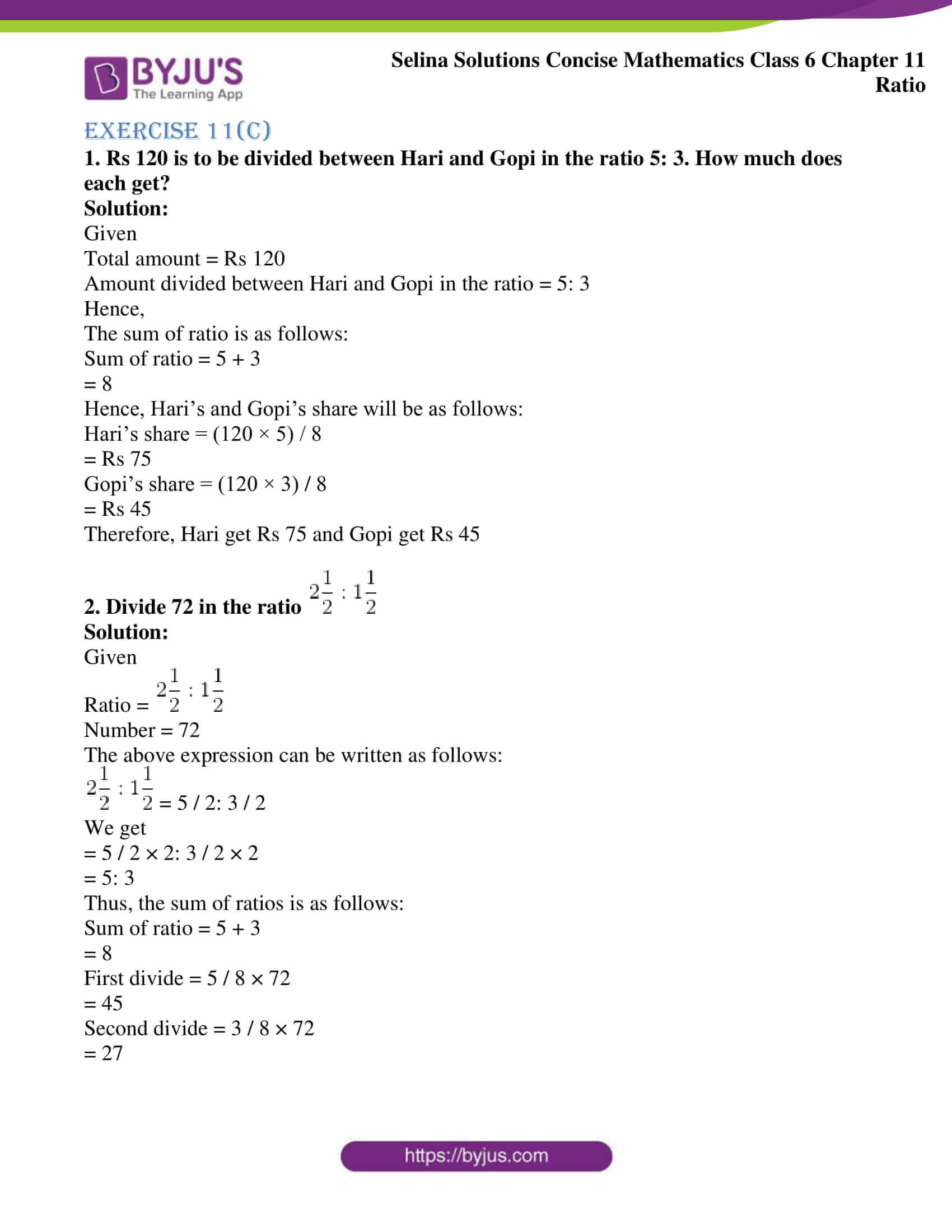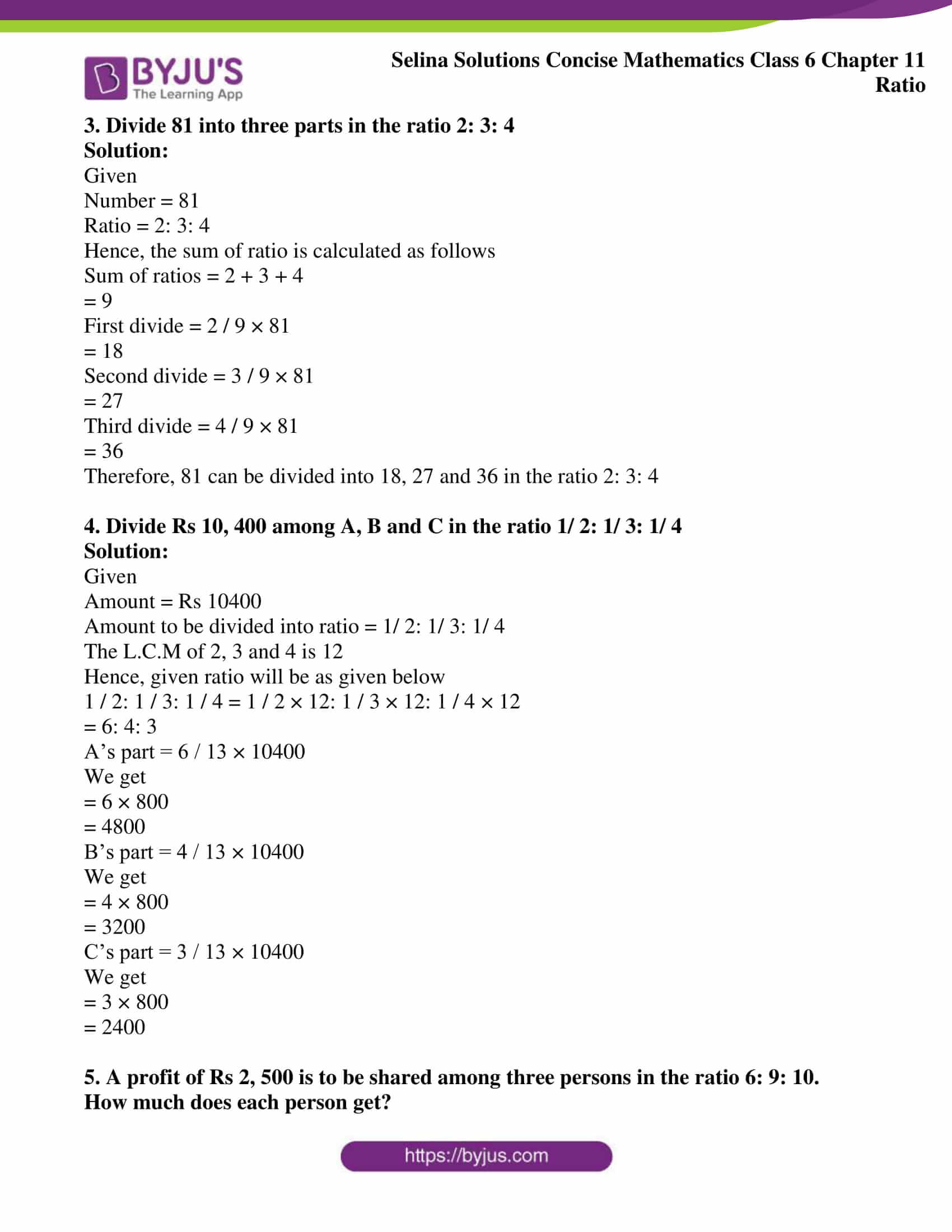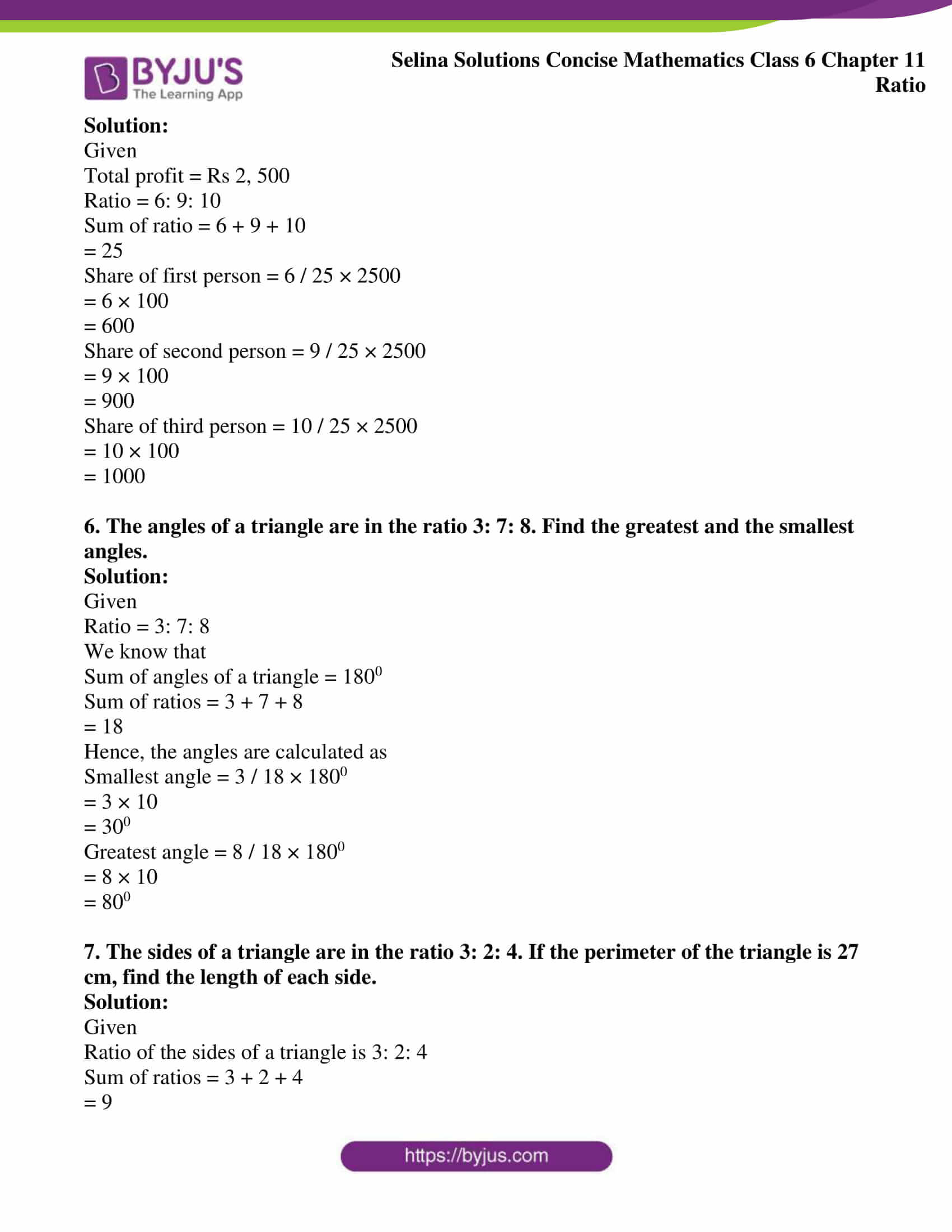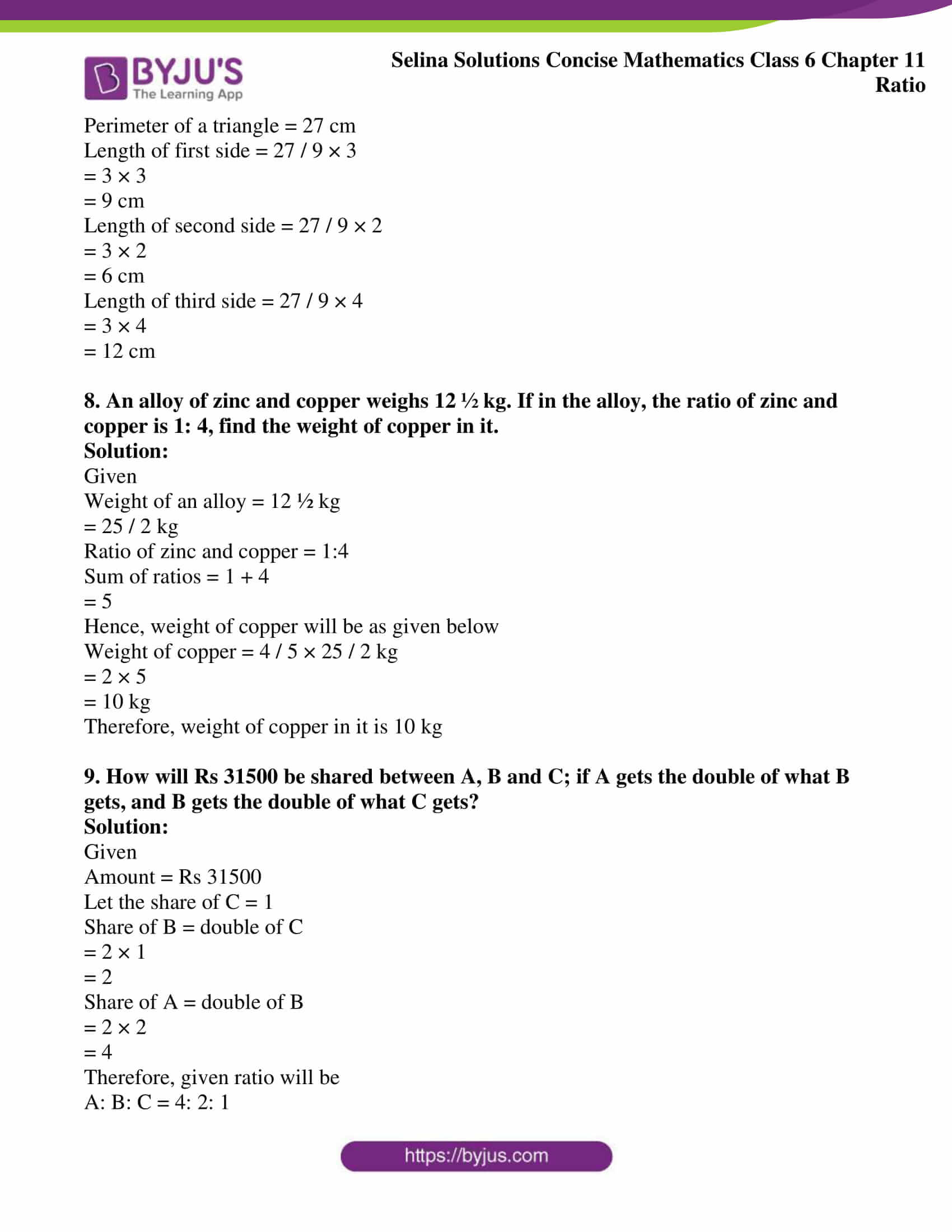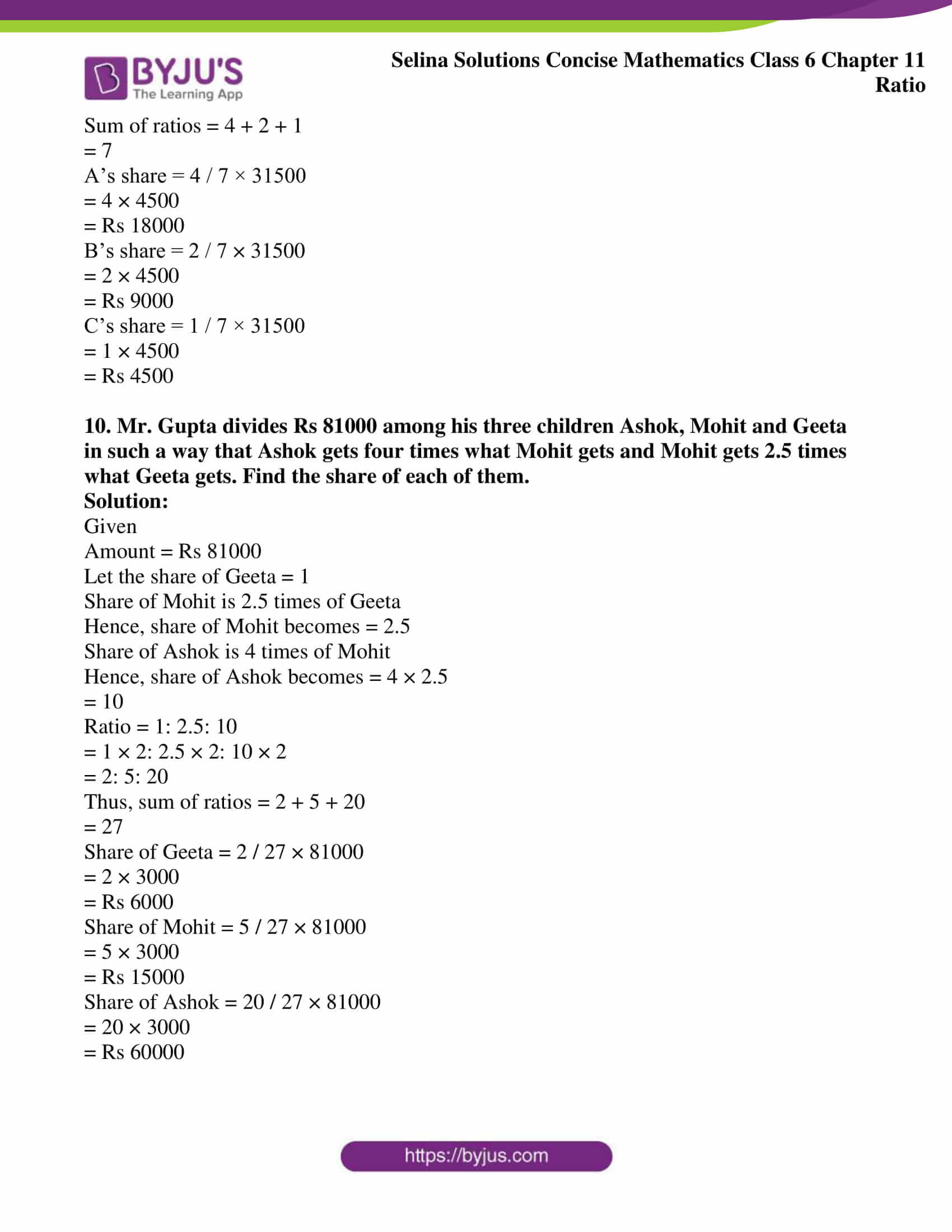### Access Selina Solutions Concise Mathematics Class 6 Chapter 11: Ratio Exercise 11(C)

#### Exercise 11(C)

1. Rs 120 is to be divided between Hari and Gopi in the ratio 5: 3. How much does each get?

Solution:

Given

Total amount = Rs 120

Amount divided between Hari and Gopi in the ratio = 5: 3

Hence,

The sum of ratio is as follows:

Sum of ratio = 5 + 3

= 8

Hence, Hari’s and Gopi’s share will be as follows:

Hari’s share = (120 × 5) / 8

= Rs 75

Gopi’s share = (120 × 3) / 8

= Rs 45

Therefore, Hari get Rs 75 and Gopi get Rs 45

2. Divide 72 in the ratio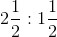Solution:

Given

Ratio =Number = 72

The above expression can be written as follows:= 5 / 2: 3 / 2

We get

= 5 / 2 × 2: 3 / 2 × 2

= 5: 3

Thus, the sum of ratios is as follows:

Sum of ratio = 5 + 3

= 8

First divide = 5 / 8 × 72

= 45

Second divide = 3 / 8 × 72

= 27

3. Divide 81 into three parts in the ratio 2: 3: 4

Solution:

Given

Number = 81

Ratio = 2: 3: 4

Hence, the sum of ratio is calculated as follows

Sum of ratios = 2 + 3 + 4

= 9

First divide = 2 / 9 × 81

= 18

Second divide = 3 / 9 × 81

= 27

Third divide = 4 / 9 × 81

= 36

Therefore, 81 can be divided into 18, 27 and 36 in the ratio 2: 3: 4

4. Divide Rs 10, 400 among A, B and C in the ratio 1/ 2: 1/ 3: 1/ 4

Solution:

Given

Amount = Rs 10400

Amount to be divided into ratio = 1/ 2: 1/ 3: 1/ 4

The L.C.M of 2, 3 and 4 is 12

Hence, given ratio will be as given below

1 / 2: 1 / 3: 1 / 4 = 1 / 2 × 12: 1 / 3 × 12: 1 / 4 × 12

= 6: 4: 3

A’s part = 6 / 13 × 10400

We get

= 6 × 800

= 4800

B’s part = 4 / 13 × 10400

We get

= 4 × 800

= 3200

C’s part = 3 / 13 × 10400

We get

= 3 × 800

= 2400

5. A profit of Rs 2, 500 is to be shared among three persons in the ratio 6: 9: 10. How much does each person get?

Solution:

Given

Total profit = Rs 2, 500

Ratio = 6: 9: 10

Sum of ratio = 6 + 9 + 10

= 25

Share of first person = 6 / 25 × 2500

= 6 × 100

= 600

Share of second person = 9 / 25 × 2500

= 9 × 100

= 900

Share of third person = 10 / 25 × 2500

= 10 × 100

= 1000

6. The angles of a triangle are in the ratio 3: 7: 8. Find the greatest and the smallest angles.

Solution:

Given

Ratio = 3: 7: 8

We know that

Sum of angles of a triangle = 1800

Sum of ratios = 3 + 7 + 8

= 18

Hence, the angles are calculated as

Smallest angle = 3 / 18 × 1800

= 3 × 10

= 300

Greatest angle = 8 / 18 × 1800

= 8 × 10

= 800

7. The sides of a triangle are in the ratio 3: 2: 4. If the perimeter of the triangle is 27 cm, find the length of each side.

Solution:

Given

Ratio of the sides of a triangle is 3: 2: 4

Sum of ratios = 3 + 2 + 4

= 9

Perimeter of a triangle = 27 cm

Length of first side = 27 / 9 × 3

= 3 × 3

= 9 cm

Length of second side = 27 / 9 × 2

= 3 × 2

= 6 cm

Length of third side = 27 / 9 × 4

= 3 × 4

= 12 cm

8. An alloy of zinc and copper weighs 12 ½ kg. If in the alloy, the ratio of zinc and copper is 1: 4, find the weight of copper in it.

Solution:

Given

Weight of an alloy = 12 ½ kg

= 25 / 2 kg

Ratio of zinc and copper = 1:4

Sum of ratios = 1 + 4

= 5

Hence, weight of copper will be as given below

Weight of copper = 4 / 5 × 25 / 2 kg

= 2 × 5

= 10 kg

Therefore, weight of copper in it is 10 kg

9. How will Rs 31500 be shared between A, B and C; if A gets the double of what B gets, and B gets the double of what C gets?

Solution:

Given

Amount = Rs 31500

Let the share of C = 1

Share of B = double of C

= 2 × 1

= 2

Share of A = double of B

= 2 × 2

= 4

Therefore, given ratio will be

A: B: C = 4: 2: 1

Sum of ratios = 4 + 2 + 1

= 7

A’s share = 4 / 7 × 31500

= 4 × 4500

= Rs 18000

B’s share = 2 / 7 × 31500

= 2 × 4500

= Rs 9000

C’s share = 1 / 7 × 31500

= 1 × 4500

= Rs 4500

10. Mr. Gupta divides Rs 81000 among his three children Ashok, Mohit and Geeta in such a way that Ashok gets four times what Mohit gets and Mohit gets 2.5 times what Geeta gets. Find the share of each of them.

Solution:

Given

Amount = Rs 81000

Let the share of Geeta = 1

Share of Mohit is 2.5 times of Geeta

Hence, share of Mohit becomes = 2.5

Share of Ashok is 4 times of Mohit

Hence, share of Ashok becomes = 4 × 2.5

= 10

Ratio = 1: 2.5: 10

= 1 × 2: 2.5 × 2: 10 × 2

= 2: 5: 20

Thus, sum of ratios = 2 + 5 + 20

= 27

Share of Geeta = 2 / 27 × 81000

= 2 × 3000

= Rs 6000

Share of Mohit = 5 / 27 × 81000

= 5 × 3000

= Rs 15000

Share of Ashok = 20 / 27 × 81000

= 20 × 3000

= Rs 60000